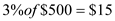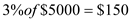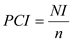# Quiz 39: The Balance of Payments, Exchange Rates, and Trade Deficits

Business

Brief introduction of the subject: DVCs have low per- capita income and IACs have high per capita income. Even if they have same growth rate, per capita income base of IACs being much higher the gap of growth between these nations increase and is never expected to converge. Given that per capita income of DVC is \$500 and that of IAC is \$5000. Growth rate of both nations iS3% With this growth rate, per capita income of DVC increases byAnd per capita income of IAC increases byTherefore change in per capita output equals \$150-\$15 i.e. \$135

Brief introduction of the subject: World Bank categorizes nations on the basis of their level of per capita of its national income. The three categories into which nations are divided on the basis of nation's per capita are:- • High-income countries • Medium-income countries • Low-income countries High income countries are those having high level of per capita income (\$37,665 in 2008). They are also known as industrially advanced countries and consist of nations such United States, Australia. Middle-income countries are those having per capita ranging from \$925 to \$11,906 (in 2008). Middle-income nations include Brazil, South Africa. Low-income countries have per capita income below \$925(in 2008). This include mainly the sub-Saharan nations.

Brief introduction of the subject: A nation's per capita income is the function of total output i.e. national income and size of its population Given that per capita income of DVC is \$300 and national income is \$3,000,000 SteP1: Formula for per capita income isHere, PCI is per capita income NI is national income n is total population Calculate size of population, nThus, Size of population iS10000 SteP2: Now, if population grows by 2% Then new population size is -SteP3: And in the same year if the total income grows by 3% Then, new total income equalsNew per capita income PCI isThus new per capita income PCI with increase in population iS303 approximately. If the population had not grown in that year then,Thus new per capita income PCI when population has not grown is \$309